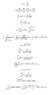# Solving Universal law of Gravitation as differential equation

• Treborman
In summary, the problem is that the differential equation for r does not have a simple form in terms of distance, and integrating does not seem to provide a straightforward answer. However, using separation of variables, one can solve the equation for t explicitly. Finally, using a named function, it is possible to solve for r implicitly as a function of time.

#### Treborman

Hi, I'm trying to solve Newton Universal law of Gravitation as a differential equation in one dimension with two objects. One mass is fixed at distance r = 0, whilst the other is some initial distance away. I'm using distance rather than displacement and also ignored the proportionality factor to make the formula simpler. So in essence, this is the differential equation I want to solve:

$$a = \frac{d^2r}{dt^2} = -\frac{1}{r^2}$$

However, I've hit a snag when trying to integrate for the 2nd time (see the image file). I have used two substitutions (a and b), and the last line in the file suggests that I will obtain the inverse function that will contain hyperbolic and polynomial terms, i.e. I won't be able to determine the original function of r with respect to t.

Can anyone help?

#### Attachments

•gravitation.JPG
14.3 KB · Views: 1,896
Last edited:
I've just discovered the Lagrange inversion theorem - would anybody be able to give me resources for this and limits if possible (preferably free, i.e. the internet).

Mult. both sides by dr/dt: Integrate.

I don't get it - do you mean integrate with respect to dr/dt? Would you be able to get me started - I was (hoping) to expand this investigation fairly quickly, not get stuck at the first hurdlewrt. both sides. Use subst.

gammamcc, I think you are missing the point. The problem is not to integrate $d^2r/dt^2= 1/r^2$. He's alread done that. That results in
$$v= \frac{dr}{dt}= \sqrt{c_1- \frac{2}{r}}$$
The question is how to integrate that.

ditto

Use separation of variables. (You wind up solving t explicitly, instead of r)Yes, of course. The whole question is about integrating
$$\int \frac{dr}{\sqrt{c_1- \frac{2}{r}}}dr$$

Your attachment was not immediately available...

This is all one expects typically. If you want more fun, put in some angular momentum.

Last edited:

$$r^{\prime \prime} = - \frac{1}{r^2}$$

times by r' and integrate to get

$$\frac{1}{2}r^{\prime 2} + C = \frac{1}{r}$$

square it to get

$$\frac{1}{r^2} = (\frac{r^{\prime 2}}{2} + C)^2$$

but we can sub r'' for the $$1/r^2$$, so

$$-r^{\prime \prime} = (\frac{r^{\prime 2}}{2} + C)^2$$

Now make the sub

$$r^{\prime} = \sqrt{2C} \tan{v}$$

to give us

$$\sqrt{2C} v^{\prime} \sec^2{v} + C^2 \sec^4{v} = 0$$

or in other words

$$v^{\prime} + \sqrt{\frac{C^3}{2}} \sec^2{v} = 0$$

now multiply by cos^2 and integrate, and you get

$$v + \frac{1}{2} \sin{2v} = \kappa - \sqrt{2 C^3} t$$

(kappa is a constant). So, what you can do is create a function, $$F$$, such that it's inverse
is defined by

$$F^{-1}(p) = p + \frac{1}{2} \sin{2p}$$

and you can express v as

$$v(t) = F(\kappa - \sqrt{2C^3}t)$$

and then

$$r(t) = \sqrt{2C} \int{ \tan{F(\kappa - \sqrt{2C^3}t)} dt}$$

or more elegently written as

$$r(t) = (2 \varphi)^{\frac{1}{3}} \int{ \tan{F(\kappa + \varphi t)} dt}$$

where $$\varphi = -\sqrt{2 C^3}$$.

...as far as I can tell... although it's late and I'm rather tired right now...

Last edited:
not trivial

This does not seem to make an explicit (or even convenient) solution for r any easier. Know this is not a trivial problem cf. Goldstein's "Classical Mechanics" pp. 98-102 (2nd ed.).

I've got a feeling it won't be possible to get a completely explicit solution for r(t). It's more a question of getting the most convenient implicit solution. One thing: the function

$$F^{-1}(p) = p + \sin{p}$$

is, as far as I can tell, one-to-one: i.e., the gradient is never negative, and there shouldn't be any ambiguity when inverting it.

I think it makes a good candidate for a named function.Last edited:
What it reduces to is an "elliptic" integral that cannt be done in terms of elementary functions. I remember seeing ("B.C."- "before computers) a 6-foot shelf of "Values of the elliptic integrals of the first and second kinds" in a university library.

I will do tihs for you:
$$\ddot{r}=-\frac{K}{r^{2}}\to\frac{1}{2}\dot{r}^{2}=\frac{K}{r}+C$$
Or, not bothering about the sign right now, or the name on the constants, we have:
$$\dot{r}=\sqrt{\frac{2K+Cr}{r}}\to\int\sqrt{\frac{r}{2K+Cr}}dr=t+A$$
Now, make the following substitution:
$$u=\sqrt{\frac{r}{2K+Cr}}\to{r}=\frac{2Ku^{2}}{1-Cu^{2}}$$
Therefore, we have:
$$\frac{dr}{du}=\frac{4Ku(1-Cu^{2})+4KCu^{3}}{(1-Cu^{2})^{2}}=\frac{4Ku}{(1-CKu^{2})^{2}}$$
Therefore, you get the integral in the equation transformed to:
$$\int\frac{4Ku^{2}du}{(1-KCu^{2})^{2}}=A+t$$
In order to perform the integration, we note that:
$$\int\frac{4Ku^{2}du}{(1-KCu^{2})^{2}}=\frac{2u}{C(1-KCu^{2})}-\frac{2}{C}\int\frac{du}{1-KCu^{2}}$$

You will therefore get an implicit equation for u as a function of t, and hence, r will also be only implicitly determined as a function of time.
The indefinite integral remaining will either be an arctan- or artanh-expression, depending on the sign of the product KC.

Last edited:
hmm maple can produce an explicit formula for r, however the formula is quite long and involves a number of "Rootof" operations. So you most likely will onlly have an eact solution for a couple of select points.

Treborman said:
Hi, I'm trying to solve Newton Universal law of Gravitation as a differential equation in one dimension with two objects. One mass is fixed at distance r = 0, whilst the other is some initial distance away. I'm using distance rather than displacement and also ignored the proportionality factor to make the formula simpler. So in essence, this is the differential equation I want to solve:

$$a = \frac{d^2r}{dt^2} = -\frac{1}{r^2}$$

However, I've hit a snag when trying to integrate for the 2nd time (see the image file). I have used two substitutions (a and b), and the last line in the file suggests that I will obtain the inverse function that will contain hyperbolic and polynomial terms, i.e. I won't be able to determine the original function of r with respect to t.

Can anyone help?
solutidn a=1/r^2 is r=b t^2/3